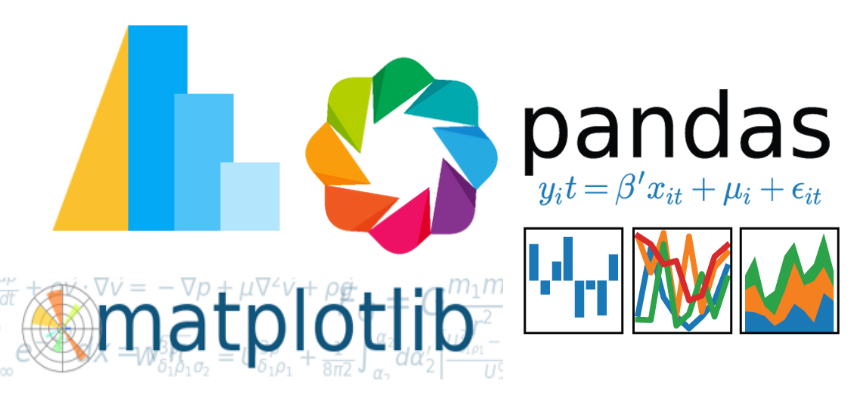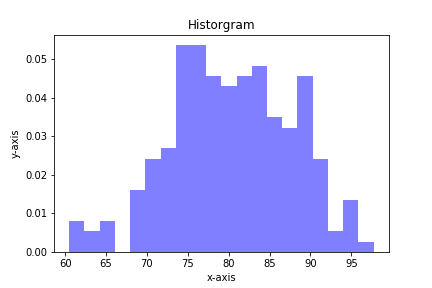## A List of Python Learning ResourcesThis post is a short list of my recommended Python learning resources. I received an email from someone who found this blog and my GitHub repo and asked about the right place to go to start learning Python. The list of resources in this post comes from the email I …

## Quiver plots using Python, matplotlib and Jupyter notebooksA quiver plot is a type of 2D plot that shows vector lines as arrows. Quiver plots are useful in electrical engineering to visualize electrical potential and valuable in mechanical engineering to show stress gradients. In this post, we will build three quiver plots using Python, matplotlib, numpy, and Jupyter notebooks.

## Plotting a stress-strain curve with four libraries: matplotlib, pandas, altair and bokehAfter watching a great webinar about plotting with different python libraries, I wanted to see what it was like to make a stress strain curve using four different modules: pandas, matplotlib, altair and bokeh (with holoviews).

## Plotting Histograms with matplotlib and PythonHistograms are a useful type of statistics plot for engineers. A histogram is a type of bar plot that shows the frequency or number of values compared to a set of value ranges. Histogram plots can be created with Python and the plotting package matplotlib. The plt.hist() function creates …

## Statistics in Python using the statistics module

In this post, we'll look at a couple of statistics functions in Python. These statistics functions are part of the Python Standard Library in the statistics module. The four functions we'll use in this post are common in statistics:

• mean - average value
• median - middle value
• mode - most often value
• standard …